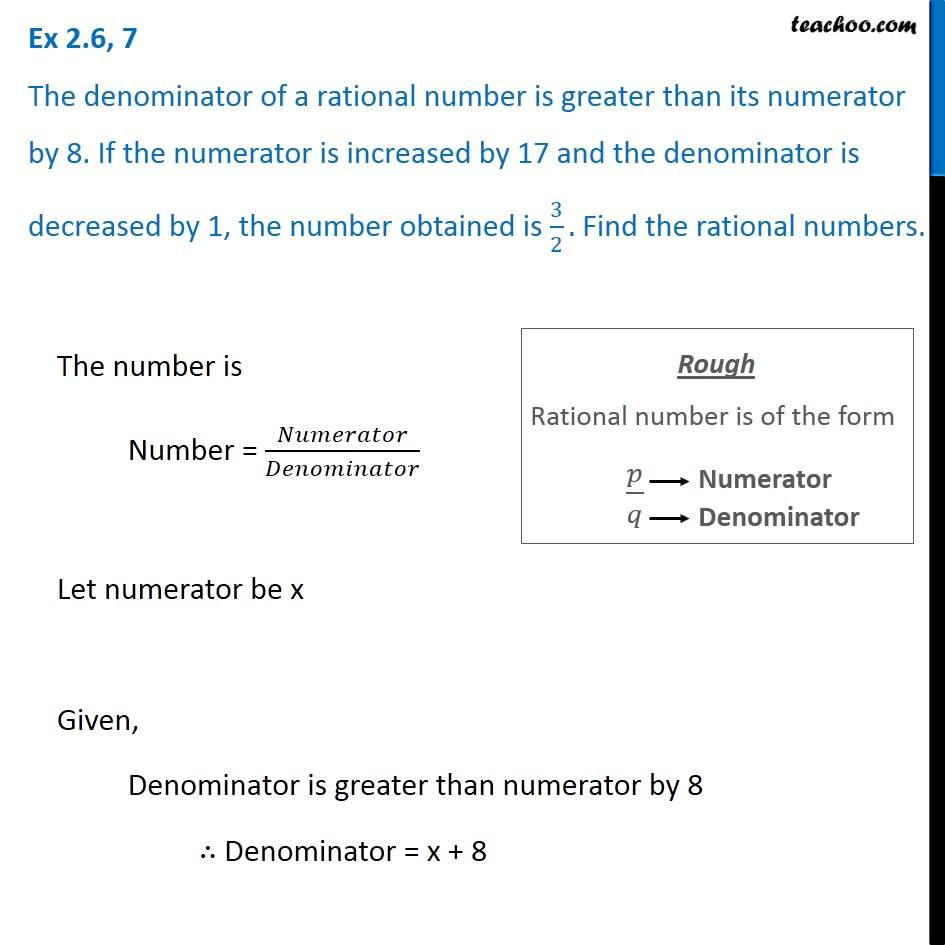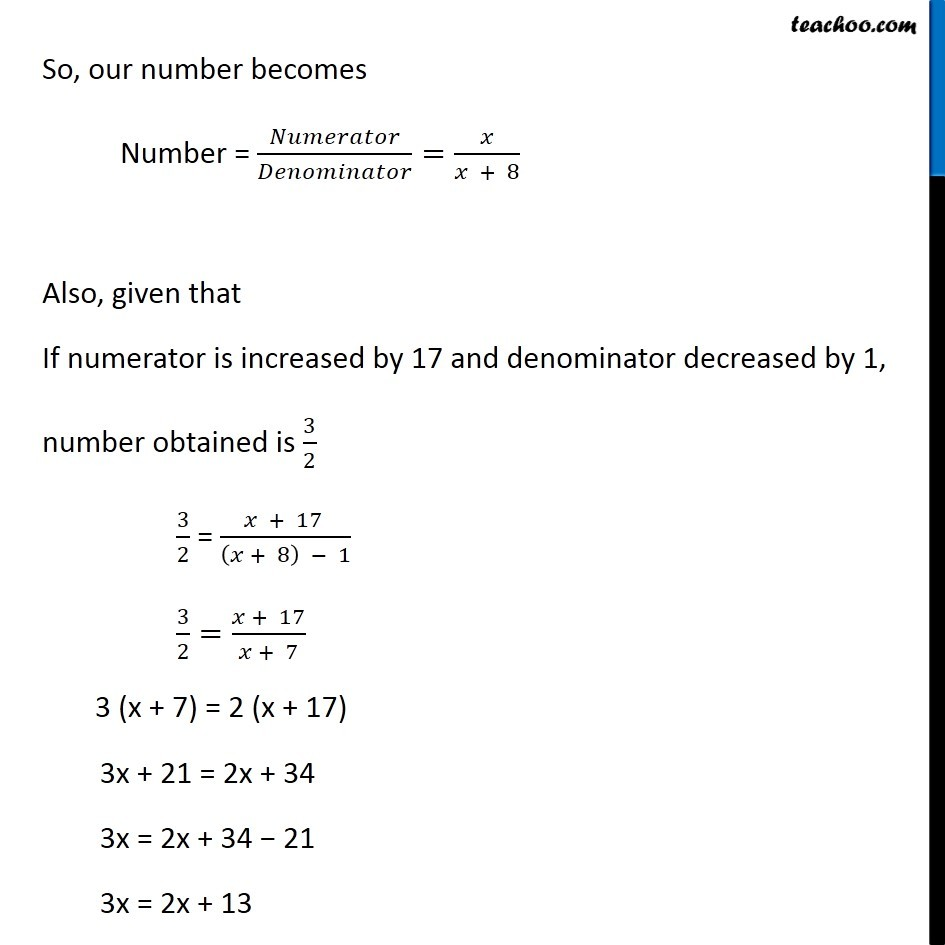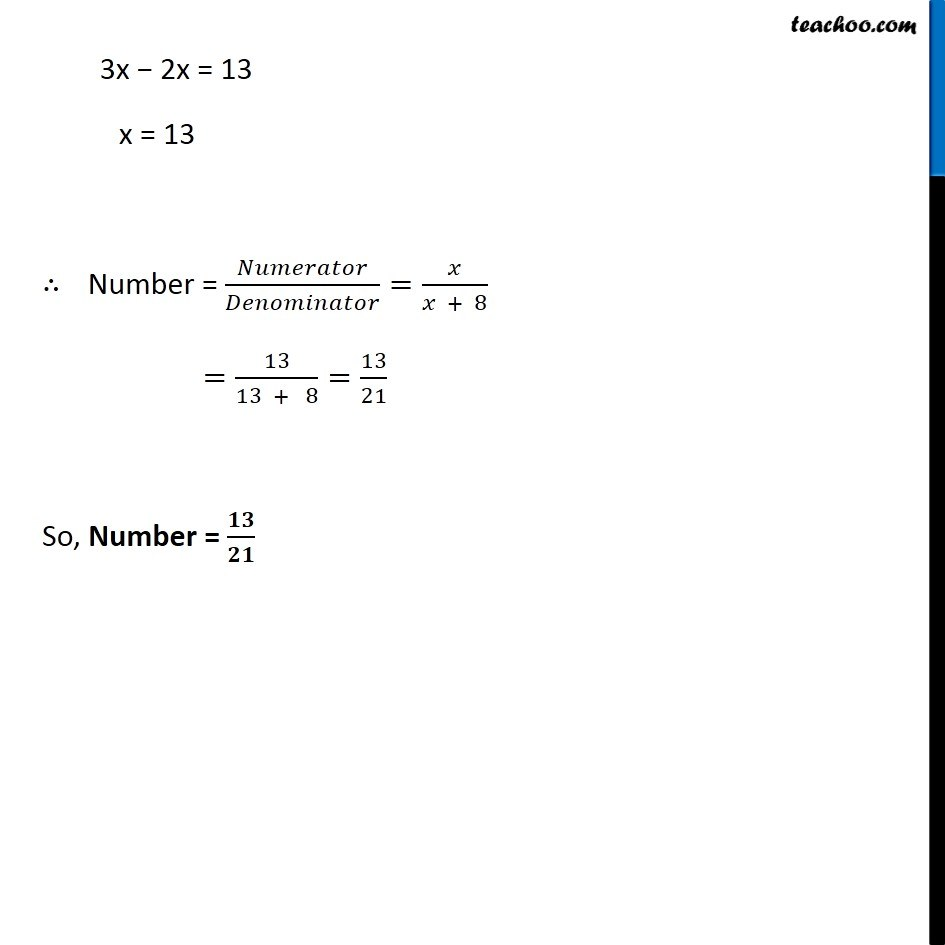Subscribe to our Youtube Channel - https://you.tube/teachoo

1. Chapter 2 Class 8 Linear Equations in One Variable
2. Concept wise
3. Forming Linear Equations - Fraction - Denominator & Numerator

Transcript

Ex 2.6, 7 The denominator of a rational number is greater than its numerator by 8. If the numerator is increased by 17 and the denominator is decreased by 1, the number obtained is 3/2. Find the rational numbers.The number is Number = Let numerator be x Given, Denominator is greater than numerator by 8 Denominator = x + 8 Rough Rational number is of the form / So, our number becomes Number = = /( + 8) Also, given that If numerator is increased by 17 and denominator decreased by 1, number obtained is 3/2 3/2 = ( + 17 )/(( + 8) 1) 3/2=( + 17)/( + 7) 3 (x + 7) = 2 (x + 17) 3x + 21 = 2x + 34 3x = 2x + 34 21 3x = 2x + 13 3x 2x = 13 x = 13 Number = = /( + 8) =13/(13 + 8)=13/21 So, Number = /

Forming Linear Equations - Fraction - Denominator & Numerator